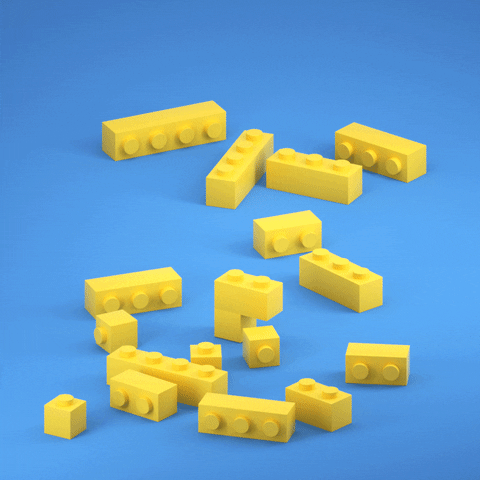# Mohamed Ali's Blog# An Introduction to Computational Thinking: The Essential Skills and Techniques for Beginners

## Introduction to Computational Thinking

Computational thinking is a problem-solving approach that involves breaking down complex problems into smaller, manageable parts and using logical reasoning, abstraction, and algorithms to find solutions. It is a fundamental skill for understanding and creating computer programs, and is increasingly relevant in many fields beyond computer science. Computational thinking allows individuals to understand and analyze data, design and test solutions, and effectively communicate and collaborate with others.

## Breaking down complex problems with abstraction

One of the key components of computational thinking is the ability to break down complex problems into smaller, more manageable parts. This process is known as abstraction. By identifying the important elements of a problem and disregarding irrelevant details, we can simplify the problem and focus on solving the most critical issues.For example, consider the problem of finding the shortest path between two points on a map. To solve this problem using computational thinking, we would first break it down into smaller parts, such as identifying the starting and ending points, creating a list of all possible paths, and calculating the distance of each path.

``````// Pseudo code for finding the shortest path

function shortestPath(start, end, map) {
let allPaths = findAllPaths(start, end, map);
let shortestPath = null;
let shortestDistance = null;
for (let i = 0; i < allPaths.length; i++) {
let distance = calculateDistance(allPaths[i]);
if (shortestPath == null || distance < shortestDistance) {
shortestPath = allPaths[i];
shortestDistance = distance;
}
}
return shortestPath;
}
``````

By abstracting the problem in this way, we can use algorithms and data structures to find the solution efficiently.

## The importance of logical reasoning in computational thinking

Logical reasoning is another essential component of computational thinking. It involves using logical statements and arguments to understand and solve problems. In programming, logical reasoning is used to create control structures that direct the flow of a program and make decisions based on specific conditions.

For example, consider a program that is designed to sort a list of numbers in ascending order. To sort the list, the program must compare each number to the next number in the list and swap them if they are in the wrong order. This process is repeated until the list is sorted.

``````// Pseudo code for sorting a list of numbers

function sortList(numbers) {
for (let i = 0; i < numbers.length; i++) {
for (let j = i+1; j < numbers.length; j++) {
if (numbers[i] > numbers[j]) {
let temp = numbers[i];
numbers[i] = numbers[j];
numbers[j] = temp;
}
}
}
return numbers;
}
``````

In this example, the program uses logical reasoning to determine when two numbers are in the wrong order and when to perform a swap. This allows the program to sort the list efficiently and effectively, which is a key aspect of computational thinking.

## The role of algorithms in computational thinking

An algorithm is a set of instructions for solving a problem or achieving a specific task. In computational thinking, algorithms are used to break down complex problems and find efficient solutions. Algorithms can be represented using pseudocode, flowcharts, or programming languages like JavaScript.

For example, consider a program that is designed to find the factorial of a given number. The factorial of a number is the product of all the positive integers less than or equal to that number. To find the factorial of a number using an algorithm, we would use a loop that starts at 1 and multiplies it by each successive integer until it reaches the given number.

``````// Pseudo code for finding the factorial of a number

function findFactorial(num) {
let result = 1;
for (let i = 1; i <= num; i++) {
result *= i;
}
return result;
}
``````

In this example, the algorithm uses a loop to iterate through the integers from 1 to the given number and multiplies each integer with the previous result to find the factorial.

## Applications of computational thinking in various fields

Computational thinking is not only relevant to computer science but also in other fields such as engineering, finance, and healthcare. Engineers use computational thinking to design and test products and systems, while financial analysts use it to analyze data and make investment decisions. In healthcare, computational thinking is used in data analysis to develop personalized medicine, discover new drugs, and improve patient outcomes.

## Developing computational thinking skills through coding education

Coding education is an effective way to develop computational thinking skills. Learning to code allows individuals to understand how computers work and how to use them to solve problems. It also teaches problem-solving, logical reasoning, and algorithmic thinking, which are all important aspects of computational thinking.

## Advancement in Computational thinking with AI and Machine learning

Artificial Intelligence (AI) and Machine Learning (ML) have significantly advanced the field of computational thinking. These technologies allow computers to learn and improve on their own, without being explicitly programmed. This has led to the development of powerful systems that can analyze large amounts of data, identify patterns, and make predictions.

## Conclusion: The future of computational thinking and its impact on society

Computational thinking is a valuable skill that is essential for understanding and creating computer programs. It is also increasingly relevant in many fields beyond computer science, and its importance will continue to grow in the future. As technology advances, computational thinking will play a vital role in solving complex problems, driving innovation, and improving our world.